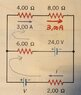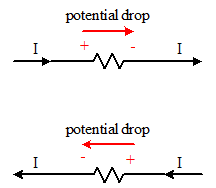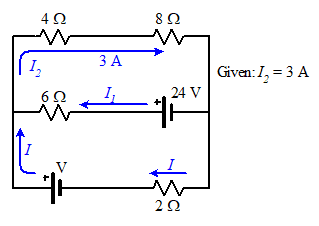# Applying Kirchhoff's laws for a loop analysis

• greg_rack
In summary, Kirchhoff's laws are applied to this one circuit, but the values for current I and voltage V are not correct.

#### greg_rack

Gold Member
Homework Statement
Calculate ##I## and ##V## in the circuit below
Relevant Equations
Kirchhoff's laws
##I=\frac{V}{R}##For this one circuit, I've applied Kirchhoff's laws as following:
$$\left\{\begin{matrix} V-6\Omega I_{6}-24V-2\Omega I_{2}=0\\ 24V-6\Omega I_{6}-12V-24V=0\\ I_{2}+I_{6}=6A \end{matrix}\right.$$
but the values I get for ##I## and ##V## aren't correct... what's wrong?

greg_rack said:
Homework Statement:: Calculate ##I## and ##V## in the circuit below
Relevant Equations:: Kirchhoff's laws
##I=\frac{V}{R}##

View attachment 271797
For this one circuit, I've applied Kirchhoff's laws as following:
$$\left\{\begin{matrix} V-6\Omega I_{6}-24V-2\Omega I_{2}=0\\ 24V-6\Omega I_{6}-12V-24V=0\\ I_{2}+I_{6}=6A \end{matrix}\right.$$
but the values I get for ##I## and ##V## aren't correct... what's wrong?

How did you get the last equation?

On your diagram, label your assumed current directions for each current you will be using in your equations. Apply those directions consistently for each equation that you write. Potential drops across resistors depend upon the direction of current flow!

Where did the 6 Amps come from in your third equation?

George Jones said:
How did you get the last equation?
I got it from second Kirchhoff's law: current entering a node is equal to the current exiting that node.
What I wrote in the thread is actually wrong, in accordance with my chose of the current directions, It would be ##I_{4}+I_{6}+I_{8}=I_{2}##.

gneill said:
Where did the 6 Amps come from in your third equation?
That is actually wrong, in accordance with what my choices are, the third equation should be: ##I_{4}+I_{6}+I_{8}=I_{2}##
By the way, with 6 amps I meant ##I_{4}+I_{8}##.

gneill said:
On your diagram, label your assumed current directions for each current you will be using in your equations. Apply those directions consistently for each equation that you write. Potential drops across resistors depend upon the direction of current flow!
Have I done something wrong? In which way would potential drops in resistors depend upon the current flow?

greg_rack said:
That is actually wrong, in accordance with what my choices are, the third equation should be: ##I_{4}+I_{6}+I_{8}=I_{2}##
By the way, with 6 amps I meant ##I_{4}+I_{8}##.
The same current flows through both the 4 and 8 Ohm resistors. They are in series so there is only one current in that branch.
Have I done something wrong? In which way would potential drops in resistors depend upon the current flow?
You need to draw in and clearly label the currents you are using before writing your equations so that you don't mistakenly change their directions (and hence potential drop directions) midway.

As for potential drops in resistors,Note how the direction of the potential drop changes with the direction of the current.

Last edited:
•greg_rack
gneill said:
The same current flows through both the 4 and 8 Ohm resistors. They are in series so there is only one current in that branch.

You need to draw in and clearly label the currents you are using before writing your equations so that you don't mistakenly change their directions (and hence potential drop directions) midway.

As for potential drops in resistors,
View attachment 271817
Note how the direction of the potential drop changes with the direction of the current.
God, I'm finding the application of these rules deceptively easy... the procedure hasn't been explained in class and I had to learn it on my own, but I'm apparently really confused about the method, in spite of its simplicity and algorithmic-nature.
Could you recommend me an exhaustive and well-made online article/video explaining how to solve any circuit with Kirchhoff? Preferably with the method I'm using, consisting of assigning a separate current to each resistor?
I think I need to dig a bit deeper into the concepts underlying the ultimate application of it.

Off hand I can't think of a particular article or video, but I do know that they are plentiful on the web if you just do a search on something like "Kirchhoff circuit analysis".

Rather than assign individual currents to each component, you want to take advantage of the fact that series-connected components will always share one current. This will minimize the number of variables that end up in your equations. For your circuit, something like this:So you work with "branch currents" rather than "component currents".

gneill said:
Off hand I can't think of a particular article or video, but I do know that they are plentiful on the web if you just do a search on something like "Kirchhoff circuit analysis".

Rather than assign individual currents to each component, you want to take advantage of the fact that series-connected components will always share one current. This will minimize the number of variables that end up in your equations. For your circuit, something like this:
View attachment 271846
So you work with "branch currents" rather than "component currents".
Got it, thank you very much! I'm confident I will soon master this topic!